Search by Topic

Resources tagged with Working systematically similar to Inside Triangles:

Filter by: Content type:
Age range:
Challenge level:

There are 321 results

Broad Topics > Using, Applying and Reasoning about Mathematics > Working systematically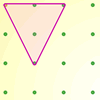Inside Triangles

Age 5 to 7 Challenge Level:

How many different triangles can you draw on the dotty grid which each have one dot in the middle?Nine-pin Triangles

Age 7 to 11 Challenge Level:

How many different triangles can you make on a circular pegboard that has nine pegs?Triangles All Around

Age 7 to 11 Challenge Level:

Can you find all the different triangles on these peg boards, and find their angles?More Transformations on a Pegboard

Age 7 to 11 Challenge Level:

Use the interactivity to find all the different right-angled triangles you can make by just moving one corner of the starting triangle.Tri.'s

Age 7 to 11 Challenge Level:

How many triangles can you make on the 3 by 3 pegboard?Triangle Animals

Age 5 to 7 Challenge Level:

How many different ways can you find to join three equilateral triangles together? Can you convince us that you have found them all?Find the Difference

Age 5 to 7 Challenge Level:

Place the numbers 1 to 6 in the circles so that each number is the difference between the two numbers just below it.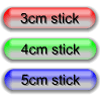Triangle Edges

Age 5 to 7 Challenge Level:

How many triangles can you make using sticks that are 3cm, 4cm and 5cm long?Four Triangles Puzzle

Age 5 to 11 Challenge Level:

Cut four triangles from a square as shown in the picture. How many different shapes can you make by fitting the four triangles back together?Mixed-up Socks

Age 5 to 7 Challenge Level:

Start with three pairs of socks. Now mix them up so that no mismatched pair is the same as another mismatched pair. Is there more than one way to do it?Egyptian Rope

Age 7 to 11 Challenge Level:

The ancient Egyptians were said to make right-angled triangles using a rope with twelve equal sections divided by knots. What other triangles could you make if you had a rope like this?Seven Flipped

Age 7 to 11 Challenge Level:

Investigate the smallest number of moves it takes to turn these mats upside-down if you can only turn exactly three at a time.Cover the Camel

Age 5 to 7 Challenge Level:

Can you cover the camel with these pieces?Tessellate the Triominoes

Age 5 to 7 Challenge Level:

What happens when you try and fit the triomino pieces into these two grids?Making Squares

Age 7 to 11

Investigate all the different squares you can make on this 5 by 5 grid by making your starting side go from the bottom left hand point. Can you find out the areas of all these squares?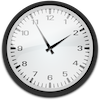How Long Does it Take?

Age 7 to 11 Challenge Level:

In this matching game, you have to decide how long different events take.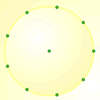Age 7 to 11 Challenge Level:

How many DIFFERENT quadrilaterals can be made by joining the dots on the 8-point circle?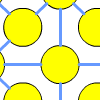One to Fifteen

Age 7 to 11 Challenge Level:

Can you put the numbers from 1 to 15 on the circles so that no consecutive numbers lie anywhere along a continuous straight line?Code Breaker

Age 7 to 11 Challenge Level:

This problem is based on a code using two different prime numbers less than 10. You'll need to multiply them together and shift the alphabet forwards by the result. Can you decipher the code?A Square of Numbers

Age 7 to 11 Challenge Level:

Can you put the numbers 1 to 8 into the circles so that the four calculations are correct?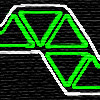Tri-five

Age 7 to 11 Challenge Level:

Find all the different shapes that can be made by joining five equilateral triangles edge to edge.How Many Times?

Age 7 to 11 Challenge Level:

On a digital 24 hour clock, at certain times, all the digits are consecutive. How many times like this are there between midnight and 7 a.m.?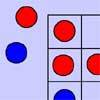Red Even

Age 7 to 11 Challenge Level:

You have 4 red and 5 blue counters. How many ways can they be placed on a 3 by 3 grid so that all the rows columns and diagonals have an even number of red counters?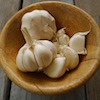Growing Garlic

Age 5 to 7 Challenge Level:

Ben and his mum are planting garlic. Use the interactivity to help you find out how many cloves of garlic they might have had.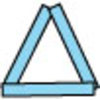Sticks and Triangles

Age 7 to 11 Challenge Level:

Using different numbers of sticks, how many different triangles are you able to make? Can you make any rules about the numbers of sticks that make the most triangles?Teddy Town

Age 5 to 14 Challenge Level:

There are nine teddies in Teddy Town - three red, three blue and three yellow. There are also nine houses, three of each colour. Can you put them on the map of Teddy Town according to the rules?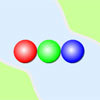Three Ball Line Up

Age 5 to 7 Challenge Level:

Use the interactivity to help get a feel for this problem and to find out all the possible ways the balls could land.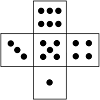Rolling That Cube

Age 5 to 11 Challenge Level:

My dice has inky marks on each face. Can you find the route it has taken? What does each face look like?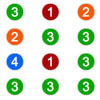Winning the Lottery

Age 7 to 11 Challenge Level:

Try out the lottery that is played in a far-away land. What is the chance of winning?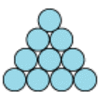Difference

Age 7 to 11 Challenge Level:

Place the numbers 1 to 10 in the circles so that each number is the difference between the two numbers just below it.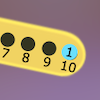Are You Well Balanced?

Age 5 to 7 Challenge Level:

Can you work out how to balance this equaliser? You can put more than one weight on a hook.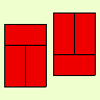Fault-free Rectangles

Age 7 to 11 Challenge Level:

Find out what a "fault-free" rectangle is and try to make some of your own.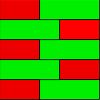Combining Cuisenaire

Age 7 to 11 Challenge Level:

Can you find all the different ways of lining up these Cuisenaire rods?Making Trains

Age 5 to 7 Challenge Level:

Can you make a train the same length as Laura's but using three differently coloured rods? Is there only one way of doing it?Same Length Trains

Age 5 to 7 Challenge Level:

How many trains can you make which are the same length as Matt's, using rods that are identical?Putting Two and Two Together

Age 7 to 11 Challenge Level:

In how many ways can you fit two of these yellow triangles together? Can you predict the number of ways two blue triangles can be fitted together?Multiples Grid

Age 7 to 11 Challenge Level:

What do the numbers shaded in blue on this hundred square have in common? What do you notice about the pink numbers? How about the shaded numbers in the other squares?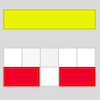Cuisenaire Counting

Age 5 to 7 Challenge Level:

Here are some rods that are different colours. How could I make a dark green rod using yellow and white rods?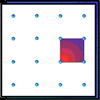Geoboards

Age 7 to 11 Challenge Level:

This practical challenge invites you to investigate the different squares you can make on a square geoboard or pegboard.Arrangements

Age 7 to 11 Challenge Level:

Is it possible to place 2 counters on the 3 by 3 grid so that there is an even number of counters in every row and every column? How about if you have 3 counters or 4 counters or....?Matching Time

Age 5 to 7 Challenge Level:

Try this matching game which will help you recognise different ways of saying the same time interval.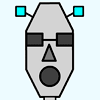Robot Monsters

Age 5 to 7 Challenge Level:

Use these head, body and leg pieces to make Robot Monsters which are different heights.Counters

Age 7 to 11 Challenge Level:

Hover your mouse over the counters to see which ones will be removed. Click to remove them. The winner is the last one to remove a counter. How you can make sure you win?Uncanny Triangles

Age 7 to 11 Challenge Level:

Can you help the children find the two triangles which have the lengths of two sides numerically equal to their areas?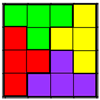Tetrafit

Age 7 to 11 Challenge Level:

A tetromino is made up of four squares joined edge to edge. Can this tetromino, together with 15 copies of itself, be used to cover an eight by eight chessboard?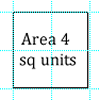Dicey Perimeter, Dicey Area

Age 7 to 11 Challenge Level:

In this game for two players, you throw two dice and find the product. How many shapes can you draw on the grid which have that area or perimeter?Junior Frogs

Age 5 to 11 Challenge Level:

Have a go at this well-known challenge. Can you swap the frogs and toads in as few slides and jumps as possible?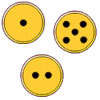Fair Exchange

Age 5 to 7 Challenge Level:

In your bank, you have three types of coins. The number of spots shows how much they are worth. Can you choose coins to exchange with the groups given to make the same total?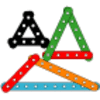Let's Investigate Triangles

Age 5 to 7 Challenge Level:

Vincent and Tara are making triangles with the class construction set. They have a pile of strips of different lengths. How many different triangles can they make?Coloured Squares

Age 5 to 7 Challenge Level:

Use the clues to colour each square.